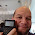## Monday, November 25, 2013

### ATAN2 using tan^-1 and angle/ARG (Various Calculators)

ATAN2

The function atan2(y,x) is defined as:

atan2(y,x) = tan^-1 (y/x) with respect to the quadrant the point (x, y) is in. In case you didn't know, with respect to point (x, y):

(x, y) is in Quadrant I if x > 0 and y > 0
(x, y) is in Quadrant II if x < 0 and y > 0
(x, y) is in Quadrant III if x < 0 and y < 0
(x, y) is in Quadrant IV if x > 0 and y < 0

Visually:This is different from the two common calculator functions used to find the arctangent: Arctangent and Argument.

The Arctangent Function

TI and Casio Calculators*: tan^-1(y/x)
Hewlett Packard Calculators*: atan (y/x)

* The majority of them

Range (Output): -90° to 90°, -π/2 to π/2 radians

How to use Arctangent to get atan(y,x)

If the point is in quadrant I:
Use atan(y/x)

If the point is in quadrant II or III:
Use atan(y/x) + 180° in degrees mode
Use atan(y/x) + π in radians mode

If the point is in quadrant IV:
Use atan(y/x) + 360° in degrees mode
Use atan(y/x) + 2*π in radians mode

Special cases have to be used x or y is equal to 0:

If x=0 and y<0, the angle is 270° (3*π/2 radians)
If x=0 and y>0, the angle is 90° (π/2 radians)
If y=0 and x<0, the angle is 180° (π radians)
If y=0 and x>0, the angle is 360° or 0° (2*π or 0 radians)

The Argument Function

The complex number x + yi is used.

TI Calculators: angle(x + y*i)
Casio and Hewlett Packard Calculators: ARG(x + y*i)

Range: -180° to 180°, -π to π radians

How to use the Argument function to get atan2(y,x)

This is a great way to get atan2, which cleverly makes the use of complex numbers. In addition, there are a lot fewer things to remember:

If y≥0 (Quadrants I and II):
Use ARG(x+yi)*

(*The angle function if you are using a TI calculator)

If y<0 (Quadrants III and IV):
Use ARG(x+yi) + 360° for degrees mode
Use ARG(x+yi) + 2*π for radians mode

I hope this tip is helpful. Happy Thanksgiving and I am very thankful for all who have read, followed, and supported my blog over the last two years.

Eddie

This blog is property of Edward Shore. 2013

1.Hi Ed,

First of all Thanks for a great blog. If the postal service will serve me well I should receive my HP Prime in the beginning of next week, and in the mean time I play with the emulator trying to learn how to program it, so I'm going through your blog learning a ton in the process.

Based on your description I made a (HP Prime) implemetaion yielding results in the range 0 to 360 or 0 to 2*Pi (depending on the angle-setting):

EXPORT ATAN2(x,y)
BEGIN
LOCAL r:=ARG(x+y*i);
IF (r < 0) THEN
IF (HAngle) THEN
r := r + 360;
ELSE
r := r + 2*π;
END;
END;
RETURN r;
END;

1.Very nice!

Eddie

2.Hello Ed, I was working on a field solution in celestial navigation for determining distance and direction with a minimum of maps, etc.

This explanation of using atan vs atan2 was perfect. Very well explained. Thank you.

Joe

3.Very helpful! Thank you very much!

4.I think this is one of the most significant information for me.And i’m glad reading your article.But should remark on some general things, the web site style is perfect,the articles is really great : D. Good job…
IWIN89

### Calculator Python: Lambda Functions

Calculator Python: Lambda Functions Introduction to Lambda Functions Lambda functions are a quick, one expression, one line, python function...